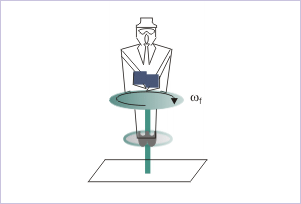# 20.6 Conservation of angular momentum (application)  (Page 2/6)

 Page 2 / 6

Solution : The system in question is composed of rigid bodies. However, internally the system can change its MI by virtue of changing positions of hands and weights held in them. The hand maneuvering, however, is internal to the system and does not affect angular momentum of the system.

Now, let us determine if there is external force and thereby external torque on the system. Force, resulting from rotation at the axis, does not constitute torque. On the other hand, we can consider the whole system as one, the weight of which acts through the center of mass. Thus, we can say that the weight of the system do not constitute torque.

We may argue that the weights of outstretched hands and weights kept in them constitute torque about the center of mass of the system. Even if we look at the system parts in this fashion, two torques acts in opposite directions due to each of the weights. They act in horizontal plane (apply right hand rule of vector product) and balance each other. We, therefore, can safely conclude that there is no external torque on the system and apply law of conservation of momentum in component form in the vertical direction.A person folds his hands drawing weights towards the axis.

If subscripts "i" and "f" correspond to initial and final values of the system, then :

$\begin{array}{l}{L}_{i}={L}_{f}\\ {I}_{i}{\omega }_{i}={I}_{f}{\omega }_{f}\end{array}$

$\begin{array}{l}⇒{\omega }_{f}=\frac{{I}_{i}{\omega }_{i}}{{I}_{f}}\end{array}$

From the question, $\phantom{\rule{2pt}{0ex}}{I}_{i}=I\phantom{\rule{2pt}{0ex}}$ and $\phantom{\rule{2pt}{0ex}}{I}_{f}=\frac{I}{2}\phantom{\rule{2pt}{0ex}}$ ,

Putting these values, we have :

$\begin{array}{l}⇒{\omega }_{f}=2{\omega }_{i}=-2\omega \end{array}$

Negative sign indicates that angular velocity is clockwise.

In order to answer the second part of the question, we need to understand the process of folding hands. We see that folding of hands has resulted in the increase of angular kinetic energy of the system as angular speed has doubled. The change in angular kinetic energy of the system is given by :

$\begin{array}{l}\Delta K=\frac{1}{2}{I}_{f}{{\omega }_{f}}^{2}-\frac{1}{2}{I}_{i}{{\omega }_{i}}^{2}\end{array}$

Putting values,

$\begin{array}{l}⇒\Delta K=\frac{1}{2}x\frac{I}{2}x{\left(2\omega \right)}^{2}-\frac{1}{2}I{\omega }^{2}=\frac{1}{2}I{\omega }^{2}\end{array}$

Note that expression of kinetic energy involves magnitude of angular velocity. Hence, sign is not considered.

Now, the basic question is “where from this kinetic energy has come?” We see here that the person is applying force on the weights on his hands. This results in doing work on a part of the system i.e. weights. Work, as we know, transfers energy from one system to another. Work, here, draws muscular energy of the hands (internal energy of the person) and transfers the same to the whole system as angular kinetic energy. As no friction is involved in the process – there is no dissipation of energy either. Further, there is no vertical elevation of the weights involved as weights are moved horizontally. As such, there is no change in gravitational potential energy of the weights. Therefore, we can conclude that the kinetic energy change due to moving weights is equal to the work done on them by muscular force in accordance with Work – angular kinetic energy theorem (in order to conserve energy of the system) :

$\begin{array}{l}⇒W=\Delta K=\frac{1}{2}I{\omega }^{2}\end{array}$

Example 2

Problem : A uniform circular platform of mass 100 kg and radius 3 m is mounted on a frictionless vertical axle and is initially stationary. A girl, weighing 60 kg, stands on the rim of the platform. She begins to walk along the rim at a speed of 1 m/s relative to the ground in clockwise direction. Is the angular momentum of the system of girl and disk-axle conserved? What is the resulting angular velocity of the platform.

#### Questions & Answers

What are the system of units
Jonah Reply
A stone propelled from a catapult with a speed of 50ms-1 attains a height of 100m. Calculate the time of flight, calculate the angle of projection, calculate the range attained
Samson Reply
58asagravitasnal firce
Amar
water boil at 100 and why
isaac Reply
what is upper limit of speed
Riya Reply
what temperature is 0 k
Riya
0k is the lower limit of the themordynamic scale which is equalt to -273 In celcius scale
Mustapha
How MKS system is the subset of SI system?
Clash Reply
which colour has the shortest wavelength in the white light spectrum
Mustapha Reply
how do we add
Jennifer Reply
if x=a-b, a=5.8cm b=3.22 cm find percentage error in x
Abhyanshu Reply
x=5.8-3.22 x=2.58
sajjad
what is the definition of resolution of forces
Atinuke Reply
what is energy?
James Reply
Ability of doing work is called energy energy neither be create nor destryoed but change in one form to an other form
Abdul
motion
Mustapha
highlights of atomic physics
Benjamin
can anyone tell who founded equations of motion !?
Ztechy Reply
n=a+b/T² find the linear express
Donsmart Reply
أوك
عباس
Quiklyyy
Sultan Reply
Moment of inertia of a bar in terms of perpendicular axis theorem
Sultan Reply
How should i know when to add/subtract the velocities and when to use the Pythagoras theorem?
Yara Reply

### Read also:

#### Get the best Physics for k-12 course in your pocket!

Source:  OpenStax, Physics for k-12. OpenStax CNX. Sep 07, 2009 Download for free at http://cnx.org/content/col10322/1.175
Google Play and the Google Play logo are trademarks of Google Inc.

Notification Switch

Would you like to follow the 'Physics for k-12' conversation and receive update notifications?By RhodesByByBy